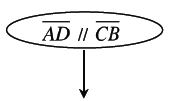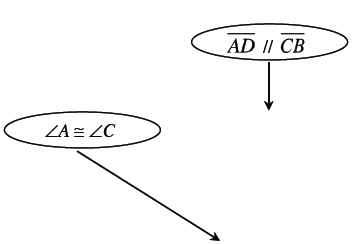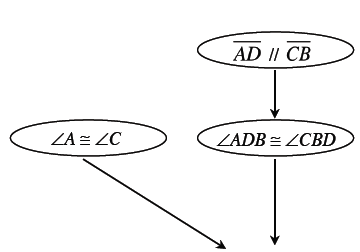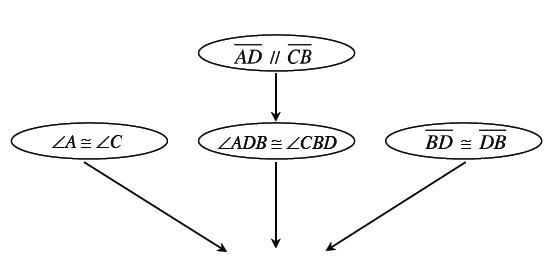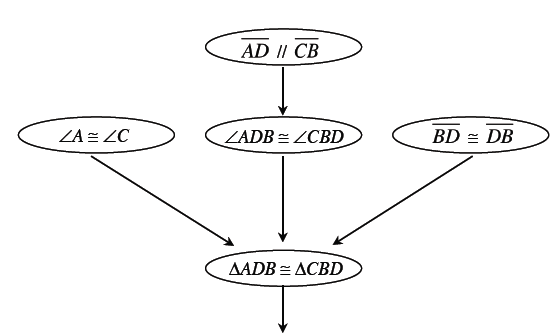### Home > CCG > Chapter Ch8 > Lesson 8.2.2 > Problem8-87

8-87.Callie started to prove that given the information in the diagram at right, then $\overline{AB} \cong \overline{CD}$. Copy her flowchart below on your paper and help her by justifying each statement.

This flowchart has 6 bubbles. Bubble # 1 has an arrow, pointing down to bubble # 3. Bubble numbers 2, 3, and 4 have arrows, pointing down to bubble # 5. Bubble # 5 has an arrow, pointing down to bubble # 6.

 Bubble # 1$\enclose{circle}{\; \\ \quad \overline{AD} \parallel \overline{CB} \quad \\}$ $\Huge \downarrow$ Bubble # 2$\enclose{circle}{\; \\ \quad \angle A \cong \angle C \quad \\}$ Bubble # 3$\enclose{circle}{\; \\ \quad \angle ADB \cong \angle CBD \quad \\}$ Bubble # 4$\enclose{circle}{\; \\ \quad \overline{BD} \cong \overline{DB} \quad \\}$ $\Huge \searrow$ $\Huge \downarrow$ $\Huge \swarrow$ Bubble # 5$\enclose{circle}{\; \\ \quad \Delta ADB \cong \Delta CBD \quad \\}$ $\Huge \downarrow$ Bubble # 6$\enclose{circle}{\; \\ \quad \overline{AB} \cong \overline{CD} \quad \\}$

Given in diagram.

Given in diagram.

If lines are parallel, then alt int angles are $\cong$.

Shared side (or Reflexive Property)

$\text{AAS}\cong$

Congruent triangles have congruent parts.